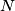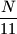### IMO Shortlist 1960 problem 1

Kvaliteta:
Avg: 2,0
Težina:
Avg: 3,0
Determine all three-digit numbers$N$ having the property that$N$ is divisible by 11, and$\dfrac{N}{11}$ is equal to the sum of the squares of the digits of$N$.
Izvor: Međunarodna matematička olimpijada, shortlist 1960